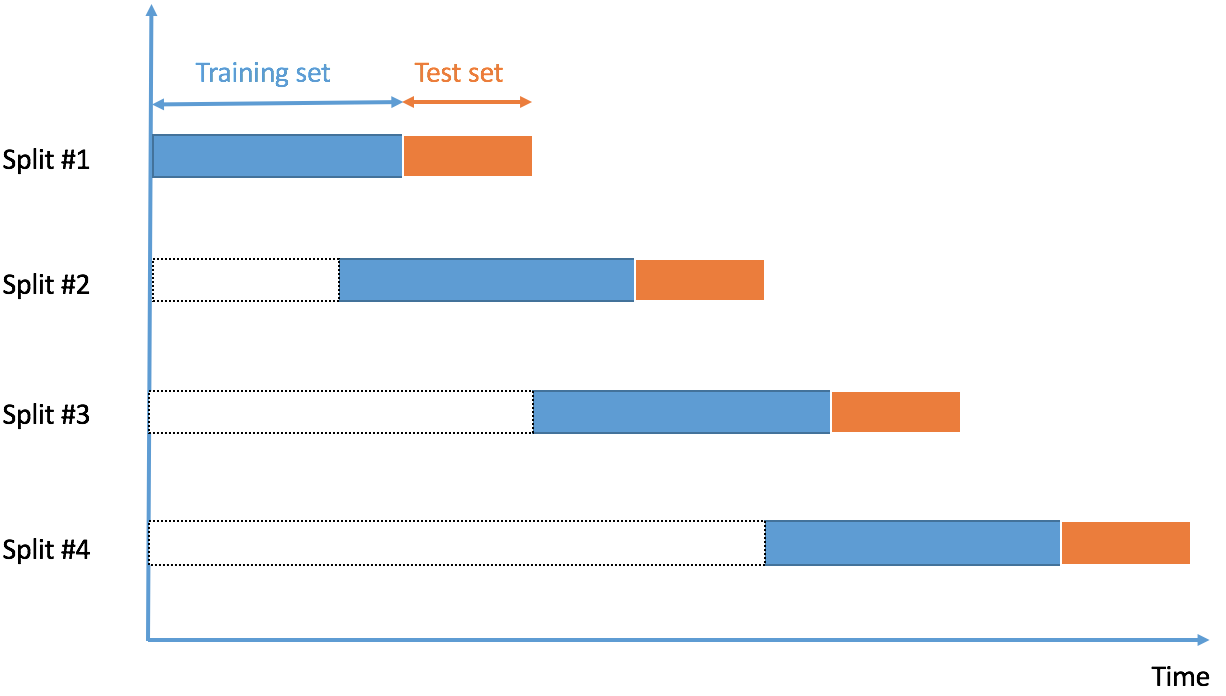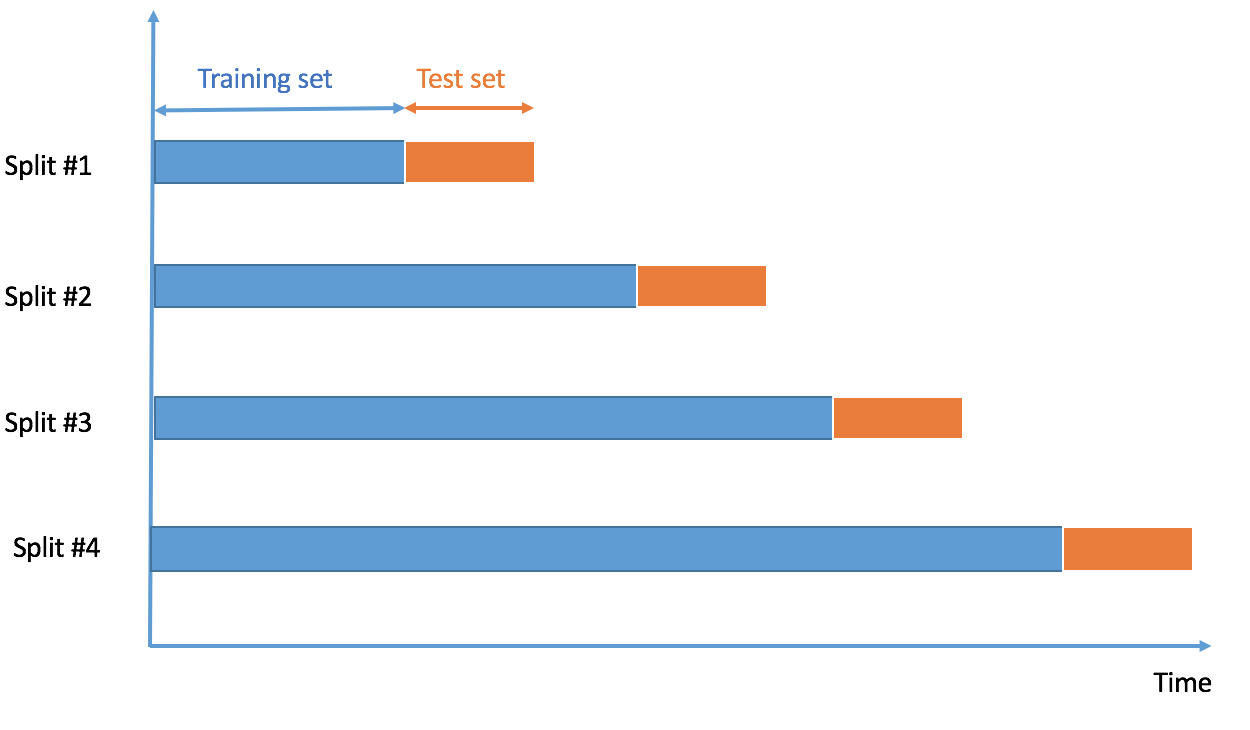Time series cross-validation is now available in crossval, using function crossval::crossval_ts. Main parameters for crossval::crossval_ts include:

• fixed_window described below in sections 1 and 2, and indicating if the training set’s size is fixed or increasing through cross-validation iterations
• initial_window: the number of points in the rolling training set
• horizon: the number of points in the rolling testing set

Yes, this type of functionality exists in packages such as caret, or forecast, but with different flavours. We start by installing crossval from its online repository (in R’s console):

library(devtools)
devtools::install_github("thierrymoudiki/crossval")
library(crossval)


## 1 - Calling crossval_ts with option fixed_window = TRUEinitial_windowis the length of the training set, depicted in blue, which is fixed through cross-validation iterations. horizon is the length of the testing set, in orange.

### 1 - 1 Using statistical learning functions

data("AirPassengers")

# regressors including trend
xreg <- cbind(1, 1:length(AirPassengers))

# cross validation with least squares regression
res <- crossval_ts(y=AirPassengers, x=xreg, fit_func = crossval::fit_lm,
predict_func = crossval::predict_lm,
initial_window = 10,
horizon = 3,
fixed_window = TRUE)

# print results
print(colMeans(res))

       ME        RMSE         MAE         MPE        MAPE
0.16473829 71.42382836 67.01472299  0.02345201  0.22106607


### 1 - 2 Using time series functions from package forecast

res <- crossval_ts(y=AirPassengers, initial_window = 10,
horizon = 3,
fcast_func = forecast::thetaf,
fixed_window = TRUE)
print(colMeans(res))

        ME         RMSE          MAE          MPE         MAPE
2.657082195 51.427170382 46.511874693  0.003423843  0.155428590


## 2 - Calling crossval_ts with option fixed_window = FALSEinitial_windowis the length of the training set, in blue, which increases through cross-validation iterations. horizon is the length of the testing set, depicted in orange.

### 2 - 1 Using statistical learning functions

# regressors including trend
xreg <- cbind(1, 1:length(AirPassengers))

# cross validation with least squares regression
res <- crossval_ts(y=AirPassengers, x=xreg, fit_func = crossval::fit_lm,
predict_func = crossval::predict_lm,
initial_window = 10,
horizon = 3,
fixed_window = FALSE)

# print results
print(colMeans(res))

     ME        RMSE         MAE         MPE        MAPE
11.35159629 40.54895772 36.07794747 -0.01723816  0.11825111


### 2 - 2 Using time series functions from package forecast

res <- crossval_ts(y=AirPassengers, initial_window = 10,
horizon = 3,
fcast_func = forecast::thetaf,
fixed_window = FALSE)
print(colMeans(res))

       ME         RMSE          MAE          MPE         MAPE
2.670281455 44.758106487 40.284267136  0.002183707  0.135572333


Note: I am currently looking for a gig. You can hire me on Malt or send me an email: thierry dot moudiki at pm dot me. I can do descriptive statistics, data preparation, feature engineering, model calibration, training and validation, and model outputs’ interpretation. I am fluent in Python, R, SQL, Microsoft Excel, Visual Basic (among others) and French. My résumé? Here!